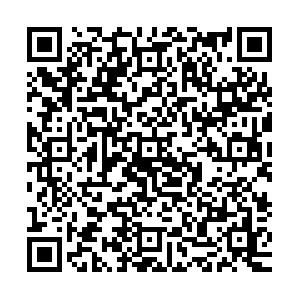# Revisiting wrong sign Yukawa coupling of type II two-Higgs-doublet model in light of recent LHC data• In light of the recently obtained LHC Higgs data, we examine the parameter space of the type II two-Higgs-doublet model, in which the 125 GeV Higgs bosons exhibit wrong sign Yukawa couplings. Combining the relevant theoretical and experimental limits, we find that the LHC Higgs data exclude most of the parameter space of the wrong sign Yukawa coupling. For $m_H=$600 GeV, the allowed samples are mainly distributed across several corners and narrow bands of $m_A<20$GeV, 30 $<m_A<120$GeV, 240 GeV $<m_A<300$GeV, 380 GeV $<m_A<430$GeV, and 480 GeV $<m_A<550$GeV. For $m_A=$600 GeV, $m_H$is required to be lower than 470 GeV. The light pseudo-scalar with a mass of 20 GeV is still permitted in the case of the wrong sign Yukawa coupling of 125 GeV Higgs bosons.
••Get Citation
Lei Wang, Hong-Xin Wang and Xiao-Fang Han. Revisiting wrong sign Yukawa coupling of type II two-Higgs-doublet model in light of the recent LHC data[J]. Chinese Physics C. doi: 10.1088/1674-1137/44/7/073101
Lei Wang, Hong-Xin Wang and Xiao-Fang Han. Revisiting wrong sign Yukawa coupling of type II two-Higgs-doublet model in light of the recent LHC data[J]. Chinese Physics C.Milestone
Article Metric

Article Views(107)
Cited by(0)
Policy on re-use
To reuse of Open Access content published by CPC, for content published under the terms of the Creative Commons Attribution 3.0 license (“CC CY”), the users don’t need to request permission to copy, distribute and display the final published version of the article and to create derivative works, subject to appropriate attribution.
###### 通讯作者: 陈斌, bchen63@163.com
• 1.

沈阳化工大学材料科学与工程学院 沈阳 110142

Title:
Email:

## Revisiting wrong sign Yukawa coupling of type II two-Higgs-doublet model in light of recent LHC data

• Department of Physics, Yantai University, Yantai 264005, China

Abstract: In light of the recently obtained LHC Higgs data, we examine the parameter space of the type II two-Higgs-doublet model, in which the 125 GeV Higgs bosons exhibit wrong sign Yukawa couplings. Combining the relevant theoretical and experimental limits, we find that the LHC Higgs data exclude most of the parameter space of the wrong sign Yukawa coupling. For $m_H=$600 GeV, the allowed samples are mainly distributed across several corners and narrow bands of $m_A<20$GeV, 30 $<m_A<120$GeV, 240 GeV $<m_A<300$GeV, 380 GeV $<m_A<430$GeV, and 480 GeV $<m_A<550$GeV. For $m_A=$600 GeV, $m_H$is required to be lower than 470 GeV. The light pseudo-scalar with a mass of 20 GeV is still permitted in the case of the wrong sign Yukawa coupling of 125 GeV Higgs bosons.

### HTML关注分享DownLoad:  Full-Size Img  PowerPoint